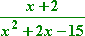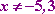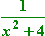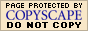Rational Expressions: Finding the Domain (page 1 of 3)

Sections: Finding the domain, Simplifying rational expressionsA "rational expression" is a polynomial fraction, and anything you could do with regular fractions you can do with rational expressions. However, since there are variables in rational expressions, there are some additional considerations.

When you dealt with fractions, you knew that the fraction could have any whole numbers for the numerator and denominator, as long as you didn't try to divide by zero. When dealing with rational expressions, you will often need to evaluate the expression, and it can be useful to know which values would cause division by zero, so you can avoid these x-values. So probably the first thing you'll do with rational expressions is find their domains.

• Find the domain of   3/x.

The domain is all values that x is allowed to be. Since I can't divide by zero (division by zero isn't allowed), I need to find all values of x that would cause division by zero. The domain will then be all other x-values. When is this denominator equal to zero? When x = 0.

Then the domain is "all x not equal to zero".

The domain doesn't care what is in the numerator of a rational expression. The domain is only influenced by the zeroes of the denominator. Will "3" ever equal zero? Of course not. Since the denominator will never equal zero, no matter what value x is, then there are no forbidden values for this rational expression, and x can be anything. So the domain is "all x".

• Give the domain of the following expression:To find the domain, I'll ignore the "x + 2" in the numerator (since the numerator does not cause division by zero) and instead I'll look at the denominator. I'll set the denominator equal to zero, and solve. The x-values in the solution will be the x-values which would cause division by zero. The domain will then be all other x-values.

x2 + 2x − 15 = 0
(x + 5)(x − 3) = 0
x = −5, x = 3

By factoring the quadratic, I found the zeroes of the denominator. The domain will then be all other x-values:• Find the domain of the following expression:
•To find the domain, I'll solve for the zeroes of the denominator:

x2 + 4 = 0
x2 = −4

This has no solution, so the denominator is never zero. Then the domain is "all x".

Top  |  1 | 2 | 3  |  Return to Index  Next >>

 Cite this article as: Stapel, Elizabeth. "Rational Expressions: Finding the Domain." Purplemath. Available from     https://www.purplemath.com/modules/rtnldefs.htm. Accessed [Date] [Month] 2016

MathHelp.com Courses
This lesson may be printed out for your personal use.